Courses
Courses for Kids
Free study material
Offline Centres
MoreLast updated date: 07th Dec 2023
Total views: 384.6k
Views today: 8.84k

# Radha made a picture of an aeroplane with coloured paper as shown in figure. Find the total area of the paper used.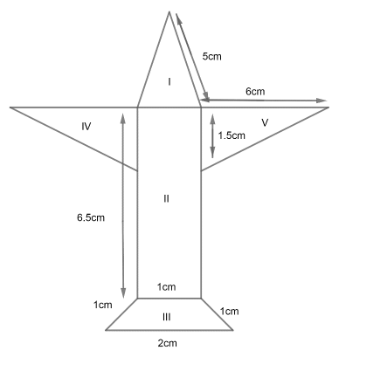Verified
384.6k+ views
Hint: Find the area of all the shapes individually and then find summation of them to get the whole area of the given aeroplane. Use the symmetric property of a given aeroplane to find all the required sides of the shapes.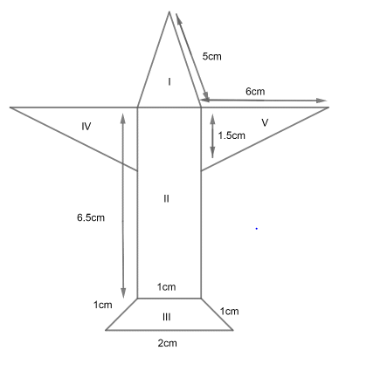Here, we have 5 shapes included in the picture of the aeroplane. Hence, we need to find the area of each shape to get the area of aeroplane.
Area of aeroplane = Area of (I + II + III + IV + V)………………… (1)
Area of shape I i.e. $\Delta ABC$ can be calculated as;
As, $\Delta ABC$ is an isosceles triangle i.e. AB = AC and length BC = IF by symmetry of the given aeroplane.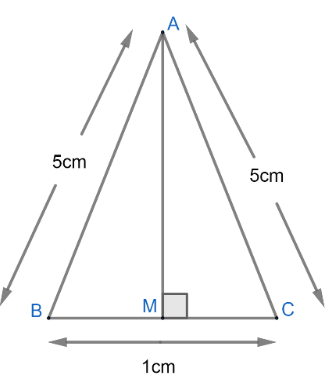Now, area of $\Delta ABC=\dfrac{1}{2}\times AM\times BC$…………………… (2)
Where AM is drawn as perpendicular to side BC.
Now, $\Delta ABM\text{ and }AMC$are congruent to each other in following way;
\begin{align} & AB=AC=5cm \\ & AM=AM\left( Common \right) \\ & \angle AMB=\angle AMC=90{}^\circ \\ \end{align}
Hence, by RHS property $\Delta ABM\cong AMC$.
So, BM = MC by CPCT.
So, now we have;
$BM=MC=\dfrac{1}{2}$
To find AM, apply Pythagoras Theorem to triangle AMC, we get
\begin{align} & A{{C}^{2}}=A{{M}^{2}}+M{{C}^{2}} \\ & {{\left( 5 \right)}^{2}}=A{{M}^{2}}{{\left( \dfrac{1}{2} \right)}^{2}} \\ & 25-\dfrac{1}{4}=A{{M}^{2}} \\ \end{align}
Or
\begin{align} & A{{M}^{2}}=\dfrac{99}{4} \\ & AM=\sqrt{\dfrac{11\times 9}{4}}=\dfrac{3}{2}\sqrt{11} \\ \end{align}
Hence, area of $\Delta ABC$from equation (2), we get;
\begin{align} & ar\left( \Delta ABC \right)=\dfrac{1}{2}\times AM\times BC \\ & =\dfrac{1}{2}\times \dfrac{3}{2}\sqrt{11}\times 1 \\ & ar\left( \Delta ABC \right)=\dfrac{3}{4}\sqrt{11}c{{m}^{2}} \\ \end{align}
Hence, area of shape I $=\dfrac{3}{4}\sqrt{11}c{{m}^{2}}$…………… (3)
Now, area of shape II can be calculated by area of rectangle i.e. length x breadth as BCFI is a rectangle.
As length of rectangle is BI = CF = 6.5cm and breadth is given as BC = IF = 1cm.
Hence,
\begin{align} & Area\left( BCFI \right)=6.5\times 1 \\ & Area\left( BCFI \right)=6.5c{{m}^{2}} \\ \end{align}
So, Area of shape II $=6.5c{{m}^{2}}$………………… (4)
As, we can observe that shape III is representing a trapezium i.e. IFGH as;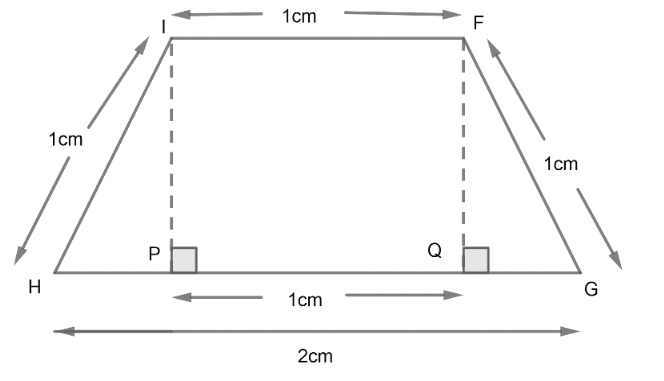Drawing IP and FQ perpendiculars to side HG. As we know area of trapezium is given by identity;
$\text{Area of trapezium =}\dfrac{1}{2}\left( \text{sum of parallel sides} \right)\times \ \text{Height}$
Or from diagram above, we get
$ar\left( IFGH \right)=\dfrac{1}{2}\left( IF+HG \right)\times IP$
As, we need to find a perpendicular IP or FQ to get an area of given trapezium. Let QG = x, so we get diagram of given trapezium, as;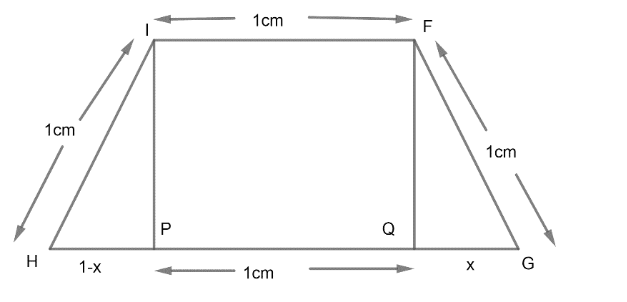Using Pythagoras Theorem to $\Delta FQG\ and\ IHP$, we get
\begin{align} & F{{G}^{2}}=F{{Q}^{2}}+Q{{G}^{2}} \\ & {{1}^{2}}=F{{Q}^{2}}+{{x}^{2}}...........................\left( 5 \right) \\ \end{align}
And,
\begin{align} & I{{H}^{2}}=H{{P}^{2}}+I{{P}^{2}} \\ & {{1}^{2}}={{\left( 1-x \right)}^{2}}+I{{P}^{2}}............\left( 6 \right) \\ \end{align}
Now, we know that FQ = IP, Hence from equations (5) and (6), we can equate values of ${{\left( FQ \right)}^{2}}\ and\ {{\left( IP \right)}^{2}}$, so we get;
\begin{align} & 1-{{x}^{2}}=1-{{\left( 1-x \right)}^{2}} \\ & 1-{{x}^{2}}=1-\left( 1+{{x}^{2}}-2x \right) \\ & -{{x}^{2}}=-1-{{x}^{2}}+2x \\ & or \\ & 2x=1 \\ & x=\dfrac{1}{2} \\ \end{align}
Hence, $QG=HP=\dfrac{1}{2}$
So, from equation (5), we get
\begin{align} & F{{Q}^{2}}=1-{{x}^{2}} \\ & F{{Q}^{2}}=1-\dfrac{1}{4}=\dfrac{3}{4} \\ & FQ=\dfrac{\sqrt{3}}{2} \\ \end{align}
Now, using identity of area of trapezium we get area of shape III be;
\begin{align} & Area\left( IFGH \right)=\dfrac{1}{2}\left( 1+2 \right)\times \dfrac{\sqrt{3}}{2} \\ & Area\left( IFGH \right)=\dfrac{3\sqrt{3}}{4}c{{m}^{2}} \\ \end{align}
Hence, area of shape III$=\dfrac{3\sqrt{3}}{4}c{{m}^{2}}$………… (7)
Now, coming to shape IV, we get;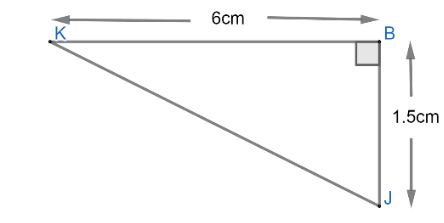As, by symmetry KB = CD = 6cm and BJ = CE = 1.5cm.
Hence, area of $\Delta KBJ$ can be given as;
\begin{align} & area\text{ }of~\left( \Delta KBJ \right)=\dfrac{1}{2}\times Base\times Height \\ & area\text{ }of~\left( \Delta KBJ \right)=\dfrac{1}{2}\times 1.5\times 6 \\ & area\text{ }of~\left( \Delta KBJ \right)==3\times 1.5 \\ & area\text{ }of~\left( \Delta KBJ \right)=4.5c{{m}^{2}} \\ \end{align}
Similarly, Area of $\Delta CDE$ should be equal to area of $\Delta KBJ$ i.e. $4.5c{{m}^{2}}$.
Hence, area of shape IV $=4.5c{{m}^{2}}$………… (8)
And
area of shape V $=4.5c{{m}^{2}}$………… (9)
Now, from the equation (1), Area of aeroplane can be given by adding all the areas of shapes calculated from equations (3), (4), (7), (8) and (9), we get;
\begin{align} & Area\text{ }of\text{ }Aeroplane=\dfrac{3}{4}\sqrt{11}+6.5+\dfrac{3\sqrt{3}}{4}+4.5+4.5 \\ & =15.5+\dfrac{3}{4}\sqrt{11}+\dfrac{3\sqrt{3}}{4}c{{m}^{2}} \\ \end{align}
As, we know
\begin{align} & \sqrt{3}=1.732 \\ & \sqrt{11}=3.316 \\ \end{align}
Hence, area of plane can be given as;
\begin{align} & Area\ of\ aeroplane=15.5+\dfrac{3}{4}\times 3.316+\dfrac{3\times 1.732}{4} \\ & Area\ of\ aeroplane=15.5+2.487+1.299 \\ & Area\ of\ aeroplane=19.286c{{m}^{2}} \\ \end{align}

Note: One can use heron’s formula for calculation of area of $\Delta ABC$.
Heron’s formula is given as;
\begin{align} & Area=\sqrt{s\left( s-a \right)\left( s-b \right)\left( s-c \right)} \\ & where\ s=\dfrac{a+b+c}{2} \\ \end{align}
And a, b, c are sides of a triangle.
Calculation is the important side of this question as well, so take care while calculating the area of all shapes provided.
One can miss any shape while calculating the area of a given aeroplane.